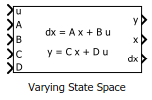# Varying State Space

State-space model with varying matrix values

•Libraries:
Control System Toolbox / Linear Parameter Varying

## Description

Use this block to implement a continuous-time state-space model with varying matrices. Feed the instantaneous values of the state matrix A, input matrix B, output matrix C, and feedforward matrix D to the corresponding input ports. The system response is given by:

`$\begin{array}{c}dx=Ax+Bu\\ y=Cx+Du,\end{array}$`

where u is the system input, y is the system output, and x and dx are the state vector and state derivatives, respectively.

Use this block and the other blocks in the Linear Parameter Varying library to implement common control elements with variable parameters or coefficients. For more information, see Model Gain-Scheduled Control Systems in Simulink.

Caution

Avoid making the C and D matrices depend on the system output y. If you have such dependence, the resulting state-space equation y = C(y)x + D(y)u creates an algebraic loop, because computing the output value y requires knowing the output value. This algebraic loop is prone to instability and divergence. Instead, try expressing C and D in terms of the time t, the block input u, and the state outputs x.

For similar reasons, avoid making A and B depend on the dx output. Note that it is safe for A and B to depend on y when y is a fixed combination of states and inputs (in other words, when y = Cx + Du, where C and D are constant matrices).

## Ports

### Input

expand all

System input signal.

State matrix of dimensions Nx-by-Nx, where Nx is the number of system states.

Input matrix of dimensions Nx-by-Nu, where Nu is the number of system inputs.

Output matrix Ny-by-Nx, where Ny is the number of system outputs.

Feedforward matrix of dimensions Ny-by-Nu.

For a zero-feedthrough state-space model, clear the Enable feedthrough checkbox. Clearing this checkbox disables the D input port. (since R2023a)

### Output

expand all

System output signal.

Current state values.

#### Dependencies

To enable this port, select the Output states parameter.

Current derivatives of the corresponding states in x.

#### Dependencies

To enable this port, select the Output state derivatives parameter.

## Parameters

expand all

Initial state values, specified as a scalar or a vector whose length is the number of system states.

To identify system states, specify state names as a:

• character vector, for a one-state plant.

• Cell array of character vectors, for a multistate plant.

Select to enable the state values output port, x.

Select to enable the state derivatives output port, dx.

Since R2023a

Enable the D input port for a state-space model with direct feedthrough. For a zero-feedthrough model, clear this checkbox. Disabling the port for zero-feedthrough models is numerically more reliable than feeding a zero-constant into the port.

## Version History

Introduced in R2017b

expand all